## How to Draw an Isosceles Triangle and Right-Angled Triangle using Protractor?

Practice Unlimited Questions

#### 1. Draw an isosceles triangle PQR in which PQ = PR, QR = 6 cm and ∠PQR = 50°.

Step 1: Draw a straight line QR 6 cm long.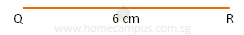Step 2: Using a protractor draw an angle of 50° from Point Q.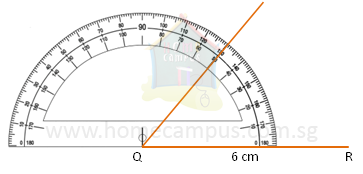Step 3: Using a protractor draw an angle of 50° from Point R.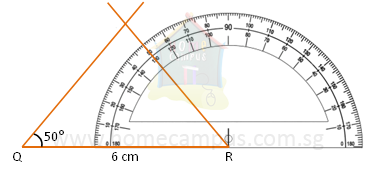The angles opposite the equal sides in an isosceles triangle are equal.
Therefore, ∠PQR = ∠PRQ = 50°.
Step 4: Mark the point where the two lines meet as P.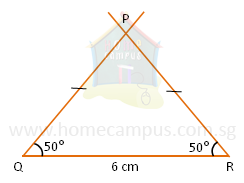ΔPQR is the required isosceles triangle.

#### 2. Draw a right-angled triangle XYZ with ∠XYZ = 90°, YZ = 5 cm and XY = 4 cm.

Step 1: Draw a straight line YZ 5cm long.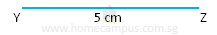Step 2: Using a protractor draw a right angle (an angle of 90°) from Point Y.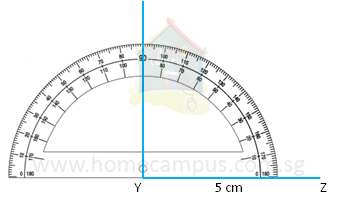Step 3: Mark the point X so that XY = 4 cm. Then, join Point X and Point Z.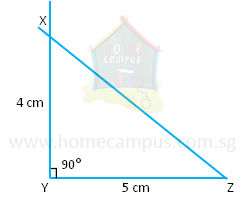Δ XYZ is the required right-angled triangle.